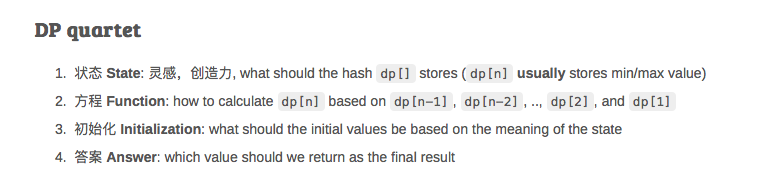## basic concept

A complex problem can be break into multiple subproblem, hope for subproblem is easy enough so that it could be easily solved. Memeorziation is part of DP which helps to reduce unnecessary computation.

## key ideas

• recursion tree: draw out for subproblem
• memoization: cache duplicate sub-steps (result of overlap subproblems)
• dynamic programing = recursion(divide & conquer) + memoization
• dp 是一种设计的思想 不是一种算法

https://stackoverflow.com/questions/12133754/whats-the-difference-between-recursion-memoization-dynamic-programming

DP can be implemented via two ways:

1. recursively (recursion + memoization)
2. itratively (forloop OR nested forloops)

Iterative algorithm can also utilize DP
iterative approach usually faster, but harder to construct.
recursive approach also call ‘lazy approach’, if ‘lazy’ not too bad, we tend to stick with it since it is easy to reasoning.

## difference to divide and conquer

• subproblems between dynmaically programming are NOT independent, solution of current subproblem usually depends on result of previous states
• on the other hand, subproblems in divide and conquer are usually independent

## top-down vs bottom up

• usually not distinguish much.

• 求最大值最小值
• 判断是否可行
• 统计方案个数

## when NOT to use DP?

• 输入数据是一个 集合 而不是 序列???
• 暴力算法的复杂度已经是多项式($n^2,n^3$)级别: 动态规划擅长与优化指数级别复杂度($2^n,n!$)到多项式级别复杂度($n^2,n^3$), 不擅长优化$n^3$到$n^2$

## DP quartet

1. 状态 State: 灵感，创造力, what should the hash dp[] stores (dp[n] usually stores min/max value)
2. 方程 Function: how to calculate dp[n] based on dp[n-1], dp[n-2], .., dp, and dp
3. 初始化 Initialization: what should the initial values be based on the meaning of the state
4. 答案 Answer: which value should we return as the final result

## DP tips:

• if given string/array have size $N$, usually we need to create a $N+1$ array, where $array$ for initialization.
• if given 2D array with size $m$ and $n$, usually we create a new $hash[m][n]$ 2D array. Try $hahs[m+1][n+1]$ if $hash[m][n]$ not work.

## DP vs Greedy Algorithm

Sometimes, DP is not necessary the most optimized solution, greedy could be more efficient than DP

https://leetcode.com/problems/jump-game/description/
Optimized Algorithm: Greedy, time complexity $O(n)$
Sub-optimized Algorithm: DP，time complexity $O(n^2)$